# [XCS224N] Lecture 4 – Backpropagation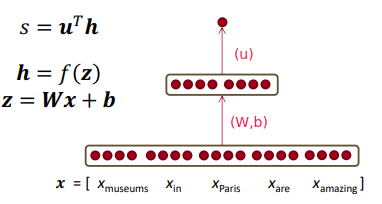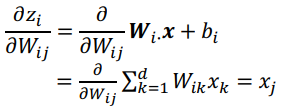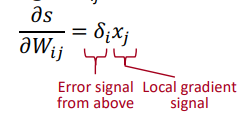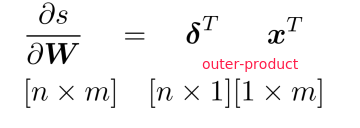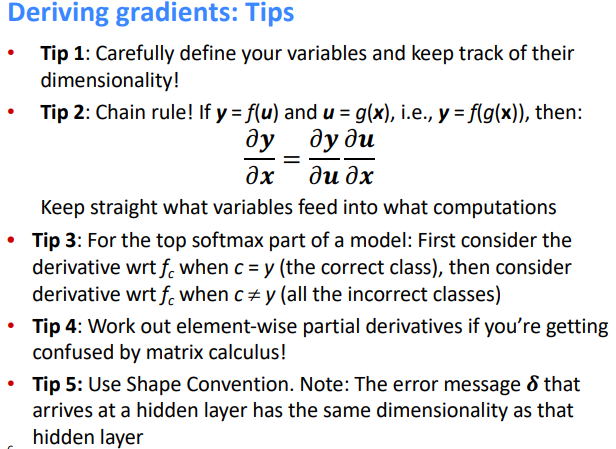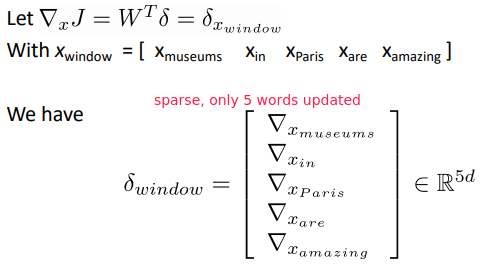pitfall in tetraining word vectors: if some word is not in training data, but other synonyms are present ⇒ only the synonyms word vectors are moved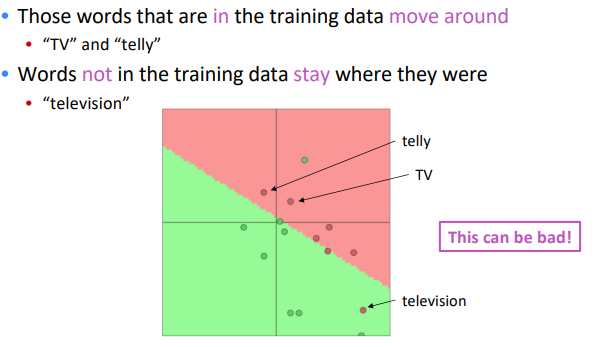takeaway: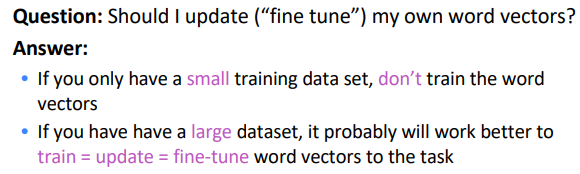## Backpropagation

backprop:

• apply (generalized) chain rule
• re-use shared stuff

### computation graph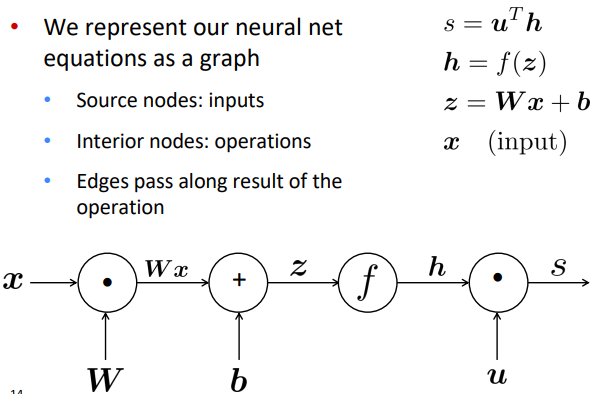⇒ Go backwards along edges, pass along gradients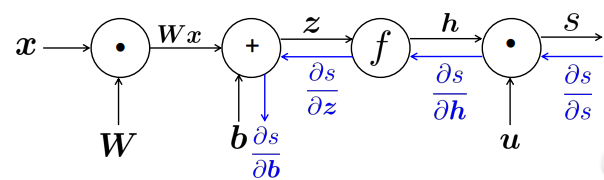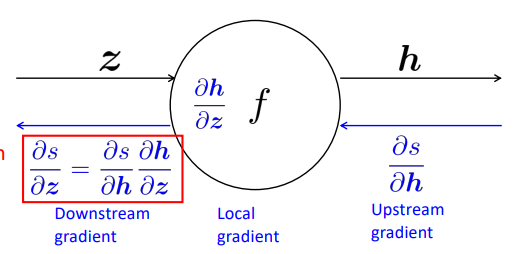for node with multiple inputs: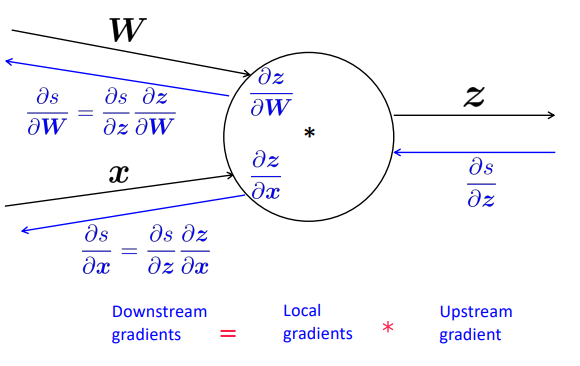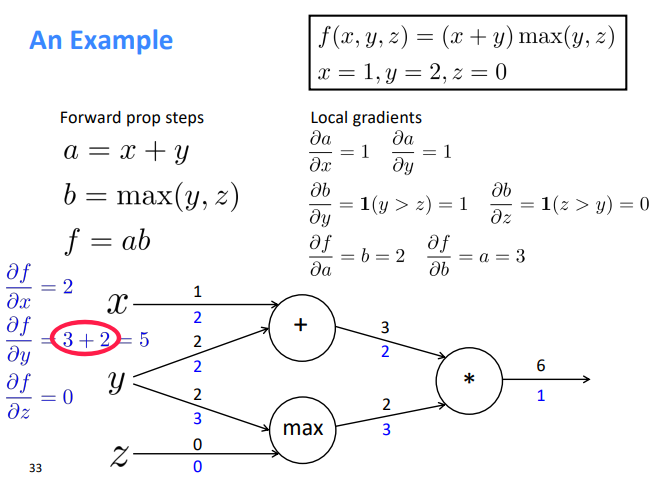## More on Backpropagation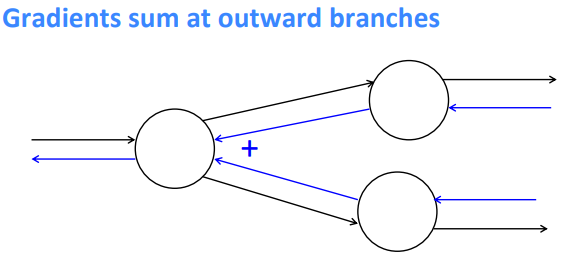intuition:

• plus( `+` ) distributes upstream grad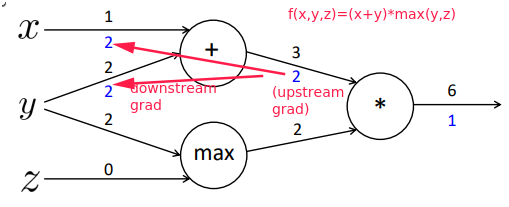• `max`routes upstream grad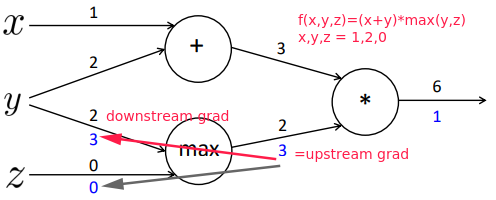• multiply( `*` ) switches the upstream grad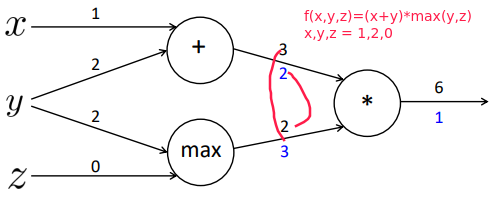efficency: compute shared part once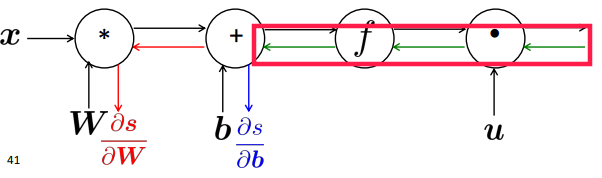### Backprop in general computation graph

comput-graph is a DAG ⇒ topological sort

• Fprop: visit nodes in topological
• Bprop: in reverse topological order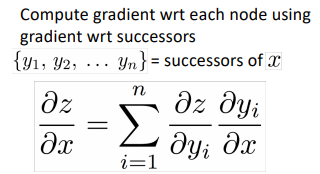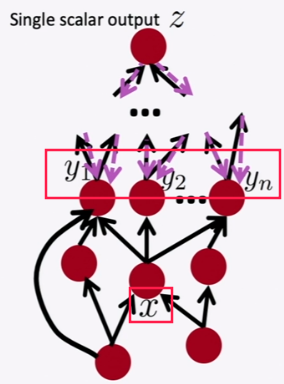Complexity = O(n) Automatic Differentiation: symbolic computation on the symbolic expression of Fprop. Moden DL framework: must provide the Fprop/Bprop formular for each node.

## Backprop Implementations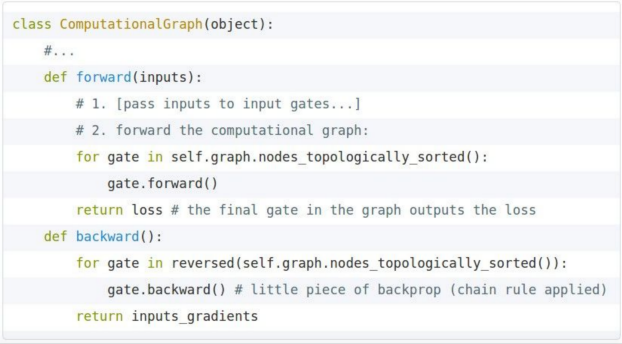for each gate, impl the forward/backward API: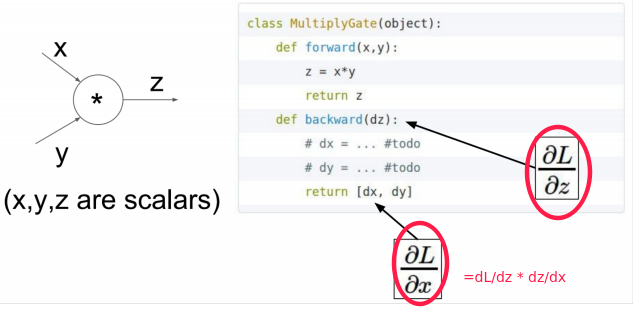Numeric Gradient For checking if the forward/backward impl is correct e.g.check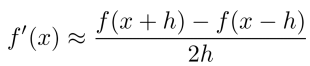## Regularization

Regularization term added to loss func to prevent overfitting: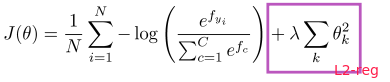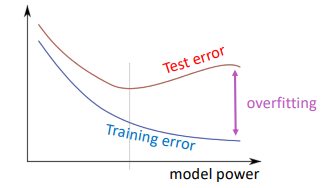## Vectorization/tensorization

avoid forloops, use matrix multiplication instead.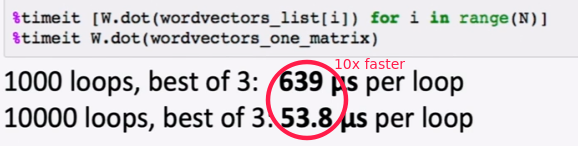## Nonlinearities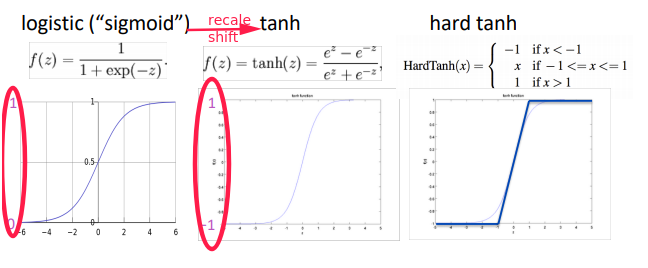tanh is recaled and shifted of sigmoid: `tanh(x) = 2 * sigmoid(2x) - 1`

new world activation func: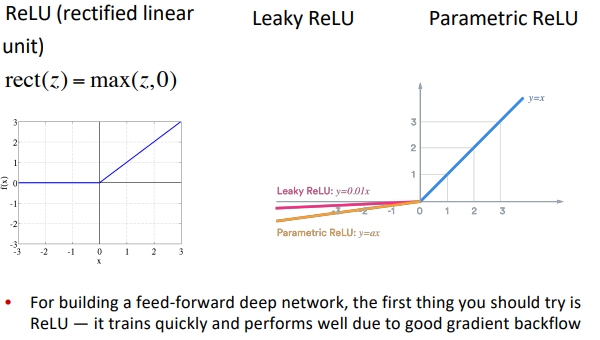## Parameter Initialization

Initialize the weights to small, random values ⇒ break the symmetry.

• Init bias to 0
• Init all other weights to Uniform(-r, r).
• Xavier initialization: variance inverse-proportional to sum of prev&next layer size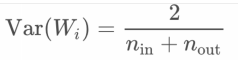## Optimizers and Learning Rates

Usually simple SGD works fine, but needs to tune the learningrate (lr). adaptive optimizers: per-parameter learning rate.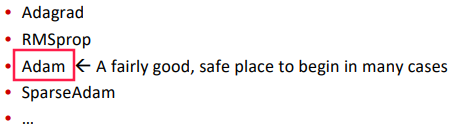learning rate:

• try with powers of 10
• learningrate-decay: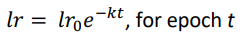(epoch = full pass over the training data)